# Weyl-Kac character formula

Weyl–Kac formula, Kac–Weyl character formula, Kac–Weyl formula, Weyl–Kac–Borcherds character formula

A formula describing the character of an irreducible highest weight module (with dominant integral highest weight) of a Kac–Moody algebra. The formula is a generalization of Weyl's classical formula for the character of an irreducible finite-dimensional representation of a semi-simple Lie algebra (cf. Character formula). The formula is very robust and has been steadily applied (with increasing technical complications) to the representations of ever wider classes of algebras, see [a3] for representations of Kac–Moody algebras and [a2] for generalized Kac–Moody (or Borcherds) algebras.

Letbe a Borcherds (colour) superalgebra (cf. also Borcherds Lie algebra) with charge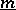and integral Borcherds–Cartan matrix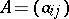, restricted with respect to the colouring matrix. (The charge counts the multiplicities of the simple roots.) Letdenote the Cartan subalgebra ofand letbe a weight-module with all weight spaces finite-dimensional. The formal character ofis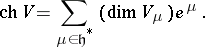For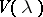an irreducible highest weight module with dominant integral highest weight, U. Ray [a6] and M. Miyamoto [a5] have established the following generalization of the Weyl–Kac–Borcherds character formula.

Letbe the Weyl group,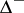the negative roots andthe set of simple roots counted with multiplicities. Let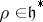be such that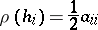for all. Define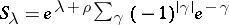, where the sum runs over all elements of the weight lattice of the form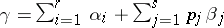such that theare distinct even imaginary roots in, the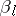are distinct odd imaginary roots in,if,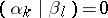for all,,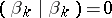if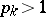, and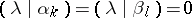for all,. Set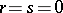if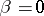, and define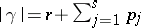. Then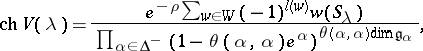whereis the colouring map induced byand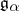is theroot space of.

In the case of Kac–Moody algebras, there are no imaginary simple roots and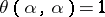for all, so one recovers the Weyl–Kac formula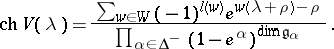These character formulas may also be applied to representations of associated quantum groups where quantum deformation theorems are known (see [a4] and [a1], for example).